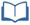# Teacher Resources

## Which Model Makes Sense?

On this page you will find lesson objectives, standards, instructional notes and UDL opportunities.

Estimated Time:  75-90 minutes

###Lesson Objectives:

• Students will gather and analyze data to determine a model of best fit (linear, quadratic or exponential).
• Students will apply their understanding of residuals and the correlation coefficient to justify the model.

Key Common Core State Standards:

S.ID.6 Represent data on two quantitative variables on a scatter plot and describe how the variables are related.

1. Fit a function to the data; use functions fitted to data to solve problems in the context of the data.
2. Informally assess the fit of a function by plotting and analyzing residuals.
3. Fit a linear function for a scatter plot that suggests a linear association.

Supporting Common Core State Standards:

F.IF.4 For a function that models a relationship between two quantities, interpret key features of graphs and tables in terms of the quantities, and sketch graphs showing key features given a verbal description of the relationship.

F.LE.1 Distinguish between situations that can be modeled with linear functions and with exponential functions.

Standards for Mathematical Practices:

MP.1 Make sense of problems and persevere in solving them.
MP.2 Reason abstractly and quantitatively.
MP.3 Construct viable arguments and critique the reasoning of others.
MP.4 Model with mathematics.
MP.5 Use appropriate tools strategically.
MP.6 Attend to precision.

## Instructional Notes:

In this lesson, students will be provided with a link to a Shodor Applet. Students also have the option to use a graphing calculator to graph scatter plots, calculate the linear regression equation, display the residual plot, and calculate the correlation coefficient.

Before students evaluate the first data set, it may be helpful to have them complete a graphic organizer comparing linear, exponential and quadratic functions.  At this point, students may not be able to identify examples of data sets that may be modeled with each of the function types. Use this as a formative assessment to check students’ current understanding of these function families.

Note about correlation coefficients: Students are required to analyze the correlation coefficient given by the calculator when performing regression for linear models only.  Discussion of the correlation coefficient as it relates to other types of regression is beyond the scope of this course.

> Go to Which Model Makes Sense? lesson

## Sample Responses

For sample responses to the Algebra II Journal questions, visit the Algebra II Journal in the Teacher Resources.

## Which Model Makes Sense - Page 8

The solution is available in the Algebra II Journal section in the Teacher Resources.  Consider providing options for students to present their findings, such as group presentations, a gallery walk, etc.

> Go to lesson, page 8

## UDL Opportunities:

Checkpoint 2.1 Clarify vocabulary and symbols.
Checkpoint 2.3 Support decoding of text, mathematical notation, and symbols.
The Student Resources section includes key vocabulary clarifications that will support the decoding of text and mathematical language.  It may be helpful to introduce some of the key terms prior to the start of this lesson.  Students will also need to be comfortable using a graphing calculator.  A quick reference guide will also be available in the Calculator Resources in the Student Resources section.

Checkpoint 3.1:  Activate or supply background knowledge.
Checkpoint 3.2:  Highlight patterns, critical features, big ideas, and relationships.
Students will need to understand key features and behaviors of linear, quadratic and simple exponential functions in order to accurately select a model to represent a given data set.   Have students create a graphic organizer (such as a Frayer Model or triple Venn diagram) to distinguish between function families.

Checkpoint 4.1:  Vary the methods for response and navigation.
Checkpoint 5:  Provide options for expression and communication.
Checkpoint 8.3 Foster collaboration and community.
Students have options to use an online applet and/or graphing calculator to analyze the data sets.  The end of lesson reflection may be submitted to the teacher.  Alternatives may include having students or groups present their findings to the class or having students do a gallery walk of final models.

Checkpoint 7.2:  Optimize relevance, value, and authenticity.
The scenarios in this lesson are based on real-world data in order to optimize relevance and authenticity.